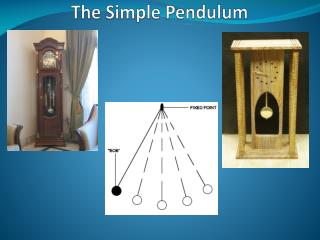DownloadDownload PresentationThe Simple Pendulum

# The Simple Pendulum

Download Presentation## The Simple Pendulum

- - - - - - - - - - - - - - - - - - - - - - - - - - - E N D - - - - - - - - - - - - - - - - - - - - - - - - - - -
##### Presentation Transcript

1. The Simple Pendulum

2. Learning Objectives Book Reference : Pages 42 The Simple Pendulum To establish which factors influence the period of a pendulum To understand how the period of a simple pendulum can be used to establish a value for g experimentally

3. During the practical last lesson, we investigated three factors which may have an effect upon the period of a simple pendulum: • Mass of the bob • Length of the string • Initial displacement (or amplitude) • Which factors did you find affected the period? • [VPL : SHM] Factors Which Affect the Period

4. Consider a simple pendulum with a bob of mass m suspended by a thread of length L. Which has a displacement s from the equilibrium position giving an angle  to the vertical Period of a Simple Pendulum 1  L s

5. The weight of the bob (mg) has the following components: • Perpendicular to motion : mg cos  • Parallel to motion : mg sin  • The restoring force F causing the SHM will be in the opposite direction: • F = -mg sin  (Using F=ma for a) Period of a Simple Pendulum 2

6. a = F/m = (-mg sin ) / m • a = -g sin  • For small values of , (< 10°), sin  = s/L • a = -g s/L • and we know that: • Acceleration = - (2f)2 x displacement • a= - (2f)2 s Period of a Simple Pendulum 3

7. a = -g s/L = - (2f)2 s (remove s and -) • g /L = (2f)2 • f = (g /L) / 2 (since T = 1/f) • T = 2 (L /g) Period of a Simple Pendulum 3

8. From the equation we can see that: • The period is independent of mass • The period is independent of initial displacement (amplitude) • The period is dependent upon the length of string • More specifically T2 is proportional to L & and a graph of T2 against L will have a gradient of 42/g • This can be used to establish a value for g Period of a Simple Pendulum 4

9. As the bob passes through the equilibrium position.... • The tension acts directly upwards and provides a centripetal force • Tension – mg = mv2/L Period of a Simple Pendulum 5

10. This work was first carried out in 1581 by Galileo when he observed lamps swinging backwards and forwards in the cathedral at Pisa Period of a Simple Pendulum Since clocks had not yet been invented he used his own pulse for timing!

11. Having conducted the experiment how could it be improved?Why are the pendulum bobs in clocks not spherical? Improving the experiment

12. Calculate the time period of a simple pendulum with lengths • 1.0m • 0.25m • Take g to be 9.81 m/s2 • Now calculate the period for the 1.0m pendulum on the moon where gravity is around 1/6th of that on Earth (g=1.6 m/s2) Problems 1